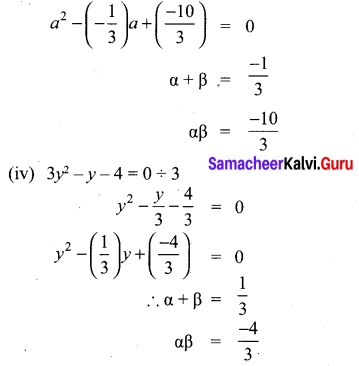# Samacheer Kalvi 10th Maths Solutions Chapter 3 Algebra Ex 3.9

## Tamilnadu Samacheer Kalvi 10th Maths Solutions Chapter 3 Algebra Ex 3.9

10th Maths Exercise 3.9 Samacheer Kalvi Question 1.
Determine the quadratic equations, whose sum and product of roots are
(i) -9, 20
(ii) $$\frac{5}{3}$$, 4
(iii) $$\frac{-3}{2}$$, -1
(iv) -(2 – a)2, (a + 5)2
Solution:
If the roots are given, general form of the quadratic equation is x2 – (sum of the roots) x + product of the roots = 0.
(i) Sum of the roots = -9
Product of the roots = 20
The equation = x2 – (-9x) + 20 = 0
⇒ x2 + 9x + 20 = 0

(ii) Sum of the roots = $$\frac{5}{3}$$
Product of the roots = 4
Required equation = x2 – (sum of the roots)x + product of the roots
= 0
⇒ x2 – $$\frac{5}{3}$$x + 4 = 0
⇒ 3x2 – 5x + 12 = 0

(iii) Sum of the roots = ($$\frac{-3}{2}$$)
(α + β) = $$\frac{-3}{2}$$
Product of the roots (αβ) = (-1)
Required equation = x2 – (α + β)x + αβ = 0
x2 – ($$\frac{-3}{2}$$)x – 1 = 0
2x2 + 3x – 2 = 0

(iv) α + β = – (2 – a)2
αβ = (a + 5)2
Required equation = x2 – (α + β)x – αβ = 0
⇒ x2 – (-(2 – a)2)x + (a + 5)2 = 0
⇒ x2 + (2 – a)2x + (a + 5)2 = 0

Ex 3.9 Class 10 Samacheer Question 2.
Find the sum and product of the roots for each of the following quadratic equations
(i) x2 + 3x – 28 = 0
(ii) x2 + 3x = 0
(iii) 3 + $$\frac{1}{a}=\frac{10}{a^{2}}$$
(iv) 3y2 – y – 4 = 0

(i) x2 + 3x – 28 = 0
(iii) 3 + $$\frac{1}{a}=\frac{10}{a^{2}}$$